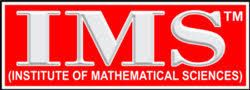# Exam Syllabus: Indian Forest Service (IFoS) Examination

## Syllabus of Paper – I

### Section-A

Linear Algebra: Vector, space, linear dependance and independance, subspaces, bases, dimensions. Finite dimensional vector spaces. Matrices, Cayley-Hamiliton theorem, eigenvalues and eigenvectors, matrix of linear transformation, row and column reduction, Echelon form, eqivalence, congruences and similarity, reduction to cannonical form, rank, orthogonal, symmetrical, skew symmetrical, unitary, hermitian, skew-hermitian formsâ€“their eigenvalues. Orthogonal and unitary reduction of quadratic and hermitian forms, positive definite quardratic forms.

Calculus: Real numbers, limits, continuity, differerentiability, mean-value theorems, Taylor’s theorem with remainders, indeterminate forms, maximas and minima, asyptotes. Functions of several variables: continuity, differentiability, partial derivatives, maxima and minima, Lagrange’s method of multipliers, Jacobian. Riemann’s definition of definite integrals, indefinite integrals, infinite and improper intergrals, beta and gamma functions. Double and triple integrals (evaluation techniques only). Areas, surface and volumes, centre of gravity.

Analytic Geometry: Cartesian and polar coordinates in two and three dimesnions, second degree equations in two and three dimensions, reduction to cannonical forms, straight lines, shortest distance between two skew lines, plane, sphere, cone, cylinder., paraboloid, ellipsoid, hyperboloid of one and two sheets and their properties.

### Section-B

Ordinary Differential Equations : Formulation of differential equations, order and degree, equations of first order and first degree, integrating factor, equations of first order but not of first degree, Clariaut’s equation, singular solution.
Higher order linear equations, with constant coefficients, complementary function and particular integral, general solution, Euler-Cauchy equation.
Second order linear equations with variable coefficients, determination of complete solution when one solution is known, method of variation of parameters.

Dynamics, Statics and Hydrostatics : Degree of freedom and constraints, rectilinerar motion, simple harmonic motion, motion in a plane, projectiles, constrained motion, work and energy, conservation of energy, motion under impulsive forces, Kepler’s laws, orbits under central forces, motion of varying mass, motion under resistance.
Equilibrium of a system of particles, work and potential energy, friction, common catenary, principle of virtual work, stability of equilibrium, equilibrium of forces in three dimensions. Pressure of heavy fluids, equilibrium of fluids under given system of forces Bernoulli’s equation, centre of pressure, thrust on curved surfaces, equilibrium of floating bodies, stability of equilibrium, metacentre, pressure of gases.

Vector Analysis : Scalar and vector fields, triple, products, differentiation of vector function of a scalar variable, Gradient, divergence and curl in cartesian, cylindrical and spherical coordinates and their physical interpretations. Higher order derivatives, vector identities and vector quations. Application to Geometry: Curves in space, curvature and torision. Serret-Frenet’s formulae, Gauss and Stokes’ theorems, Green’s identities.

## Syllabus of Paper – II

### Section-A

Algebra: Groups, subgroups, normal subgroups, homomorphism of groups quotient groups basic isomorophism theorems, Sylow’s group, permutation groups, Cayley theorem. Rings and ideals, principal ideal domains, unique factorization domains and Euclidean domains. Field extensions, finite fields.

Real Analysis : Real number system, ordered sets, bounds, ordered field, real number system as an ordered field with least upper bound property, cauchy sequence, completeness, Continuity and uniform continuity of functions, properties of continuous functions on compact sets. Riemann integral, improper integrals, absolute and conditional convergence of series of real and complex terms, rearrangement of series. Uniform convergence, continuity, differentiability and integrability for sequences and series of functions. Differentiation of fuctions of several variables, change in the order of partial derivatives, implict function theorem, maxima and minima. Multiple integrals. Complex Analysis : Analytic function, Cauchy-Riemann equations, Cauchy’s theorem, Cauchy’s integral formula, power series, Taylor’s series, Laurent’s Series, Singularities, Cauchy’s residue theorem, contour integration. Conformal mapping, bilinear transformations.

Linear Programming : Linear programming problems, basic solution, basic feasible solution and optimal solution, graphical method and Simplex method of solutions. Duality.
Transportation and assignment problems. Travelling salesman problmes.

### Section-B

Partial differential equations: Curves and surfaces in three dimesnions, formulation of partial differential equations, solutions of equations of type dx/p=dy/q=dz/r; orthogonal trajectories, pfaffian differential equations; partial differential equations of the first order, solution by Cauchy’s method of characteristics; Charpit’s method of solutions, linear partial differential equations of the second order with constant coefficients, equations of vibrating string, heat equation, laplace equation.

Numerical Analysis and Computer programming: Numerical methods: Solution of algebraic and transcendental equations of one variable by bisection, Regula-Falsi and Newton-Raphson methods, solution of system of linear equations by Gaussian elimination and Gauss-Jordan (direct) methods, Gauss-Seidel(iterative) method. Newton’s (Forward and backward) and Lagrange’s method of interpolation. Numerical integration: Simpson’s one-third rule, tranpezodial rule, Gaussian quardrature formula. Numerical solution of ordinary differential equations: Euler and Runge Kutta-methods.

Computer Programming: Storage of numbers in Computers, bits, bytes and words, binary system. arithmetic and logical operations on numbers. Bitwise operations. AND, OR , XOR, NOT, and shift/rotate operators. Octal and Hexadecimal Systems. Conversion to and form decimal Systems. Representation of unsigned integers, signed integers and reals, double precision reals and long integrers. Algorithms and flow charts for solving numerical analysis problems. Developing simple programs in Basic for problems involving techniques covered in the numerical analysis.

Mechanics and Fluid Dynamics : Generalised coordinates, constraints, holonomic and non-holonomic , systems. D’ Alembert’s principle and Lagrange’ equations, Hamilton equations, moment of intertia, motion of rigid bodies in two dimensions. Equation of continuity, Euler’s equation of motion for inviscid flow, stream-lines, path of a particle, potential flow, two-dimensional and axisymetric motion, sources and sinks, vortex motion, flow past a cylinder and a sphere, method of images. Navier-Stokes equation for a viscous fluid.